Publicité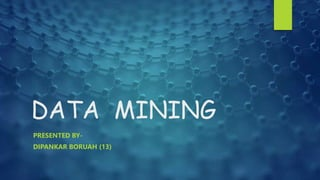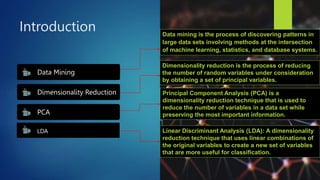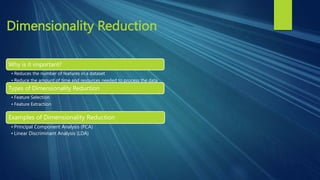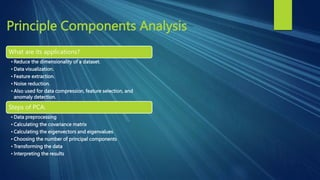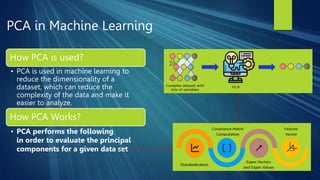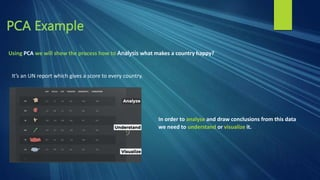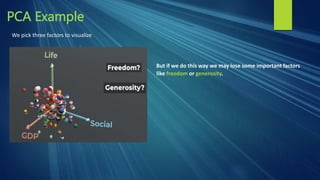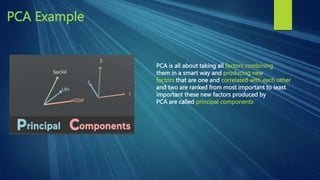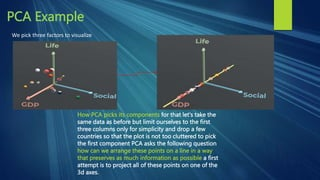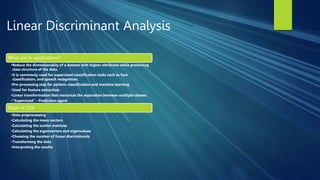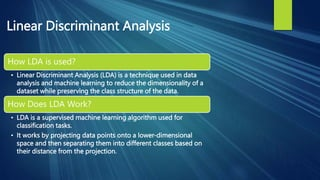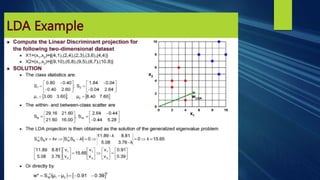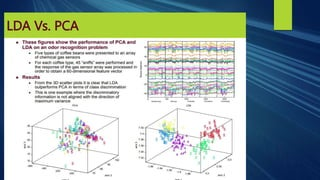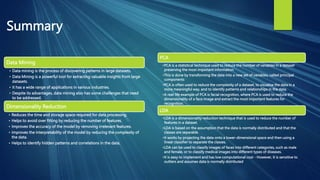1 sur 18
Publicité

### DATA MINING.pptx

1. DATA MINING PRESENTED BY- DIPANKAR BORUAH (13)
2. Introduction Data Mining Dimensionality Reduction PCA LDA Data mining is the process of discovering patterns in large data sets involving methods at the intersection of machine learning, statistics, and database systems. Dimensionality reduction is the process of reducing the number of random variables under consideration by obtaining a set of principal variables. Principal Component Analysis (PCA) is a dimensionality reduction technique that is used to reduce the number of variables in a data set while preserving the most important information. Linear Discriminant Analysis (LDA): A dimensionality reduction technique that uses linear combinations of the original variables to create a new set of variables that are more useful for classification.
3. Dimensionality Reduction Why is it important? • Reduces the number of features in a dataset • Reduce the amount of time and resources needed to process the data Types of Dimensionality Reduction • Feature Selection • Feature Extraction Examples of Dimensionality Reduction • Principal Component Analysis (PCA) • Linear Discriminant Analysis (LDA)
4. Principle Components Analysis What are its applications? • Reduce the dimensionality of a dataset. • Data visualization. • Feature extraction. • Noise reduction. • Also used for data compression, feature selection, and anomaly detection. Steps of PCA: • Data preprocessing • Calculating the covariance matrix • Calculating the eigenvectors and eigenvalues • Choosing the number of principal components • Transforming the data • Interpreting the results
5. PCA in Machine Learning How PCA is used? • PCA is used in machine learning to reduce the dimensionality of a dataset, which can reduce the complexity of the data and make it easier to analyze. How PCA Works? • PCA performs the following in order to evaluate the principal components for a given data set
6. PCA Example Using PCA we will show the process how to Analysis what makes a country happy? It’s an UN report which gives a score to every country. In order to analyse and draw conclusions from this data we need to understand or visualize it.
7. PCA Example We pick three factors to visualize But if we do this way we may lose some important factors like freedom or generosity.
8. PCA Example PCA is all about taking all factors combining them in a smart way and producing new factors that are one and correlated with each other and two are ranked from most important to least important these new factors produced by PCA are called principal components
9. PCA Example And they are constructed in such a way that if you restrict your attention to the first few components only you would still get a fateful representation of the data.
10. PCA Example We pick three factors to visualize But if we do this way we may lose some important factors like freedom or generosity.
11. PCA Example We pick three factors to visualize But if we do this way we may lose some important factors like freedom or generosity.
12. PCA Example We pick three factors to visualize How PCA picks its components for that let's take the same data as before but limit ourselves to the first three columns only for simplicity and drop a few countries so that the plot is not too cluttered to pick the first component PCA asks the following question how can we arrange these points on a line in a way that preserves as much information as possible a first attempt is to project all of these points on one of the 3d axes.
13. Linear Discriminant Analysis What are its applications? •Reduce the dimensionality of a dataset with higher attributes while preserving class structure of the data. •It is commonly used for supervised classification tasks such as face classification, and speech recognition. •Pre-processing step for pattern-classification and machine learning •Used for feature extraction. •Linear transformation that maximize the separation between multiple classes. •“Supervised” - Prediction agent Steps of LDA: •Data preprocessing •Calculating the mean vectors •Calculating the scatter matrices •Calculating the eigenvectors and eigenvalues •Choosing the number of linear discriminants •Transforming the data •Interpreting the results.
14. Linear Discriminant Analysis How LDA is used? • Linear Discriminant Analysis (LDA) is a technique used in data analysis and machine learning to reduce the dimensionality of a dataset while preserving the class structure of the data. How Does LDA Work? • LDA is a supervised machine learning algorithm used for classification tasks. • It works by projecting data points onto a lower-dimensional space and then separating them into different classes based on their distance from the projection.
15. LDA Example
16. LDA Vs. PCA
17. Summary Data Mining • Data mining is the process of discovering patterns in large datasets. • Data Mining is a powerful tool for extracting valuable insights from large datasets. • It has a wide range of applications in various industries. • Despite its advantages, data mining also has some challenges that need to be addressed. Dimensionality Reduction • Reduces the time and storage space required for data processing. • Helps to avoid over fitting by reducing the number of features. • Improves the accuracy of the model by removing irrelevant features. • Improves the interpretability of the model by reducing the complexity of the data. • Helps to identify hidden patterns and correlations in the data. PCA •PCA is a statistical technique used to reduce the number of variables in a dataset preserving the most important information. •This is done by transforming the data into a new set of variables, called principal components •PCA is often used to reduce the complexity of a dataset, to visualize the data in a more meaningful way, and to identify patterns and relationships in the data. •A real-life example of PCA is facial recognition, where PCA is used to reduce the dimensionality of a face image and extract the most important features for recognition. LDA •LDA is a dimensionality reduction technique that is used to reduce the number of features in a dataset. •LDA is based on the assumption that the data is normally distributed and that the classes are separable. •It works by projecting the data onto a lower-dimensional space and then using a linear classifier to separate the classes. •LDA can be used to classify images of faces into different categories, such as male and female, or to classify medical images into different types of diseases. •It is easy to implement and has low computational cost - However, it is sensitive to outliers and assumes data is normally distributed
18. Thank You!
Publicité